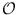Next: Strongly Connected Components (flexible) Up: Graphs and Iterators Previous: Depth First Search (flexible)   Contents   Index

# Topological Sort (flexible) ( GIT_TOPOSORT )

Definition

An instance algorithm of class GIT_TOPOSORT< OutAdjIt, Indeg, Queuetype > is an implementation of an algorithm that iterates over all nodes in some topological order, if the underlying graph is acyclic. An object of this class maintains an internal queue, which contains all nodes (in form of adjacency iterators where the current node is equal to the fixed node) that are not yet passed, but all its predecessors have been passed.

Iterator version: There is an iterator version of this algorithm: TOPO_It. Usage is similar to that of node iterators without the ability to go backward in the sequence and only a graph is allowed at creation time. Additionally there is TOPO_rev_It which traverses the graph in reversed topological order.

#include < LEDA/graph/graph_iterator.h >

Creation

 GIT_TOPOSORT< OutAdjIt, Indeg, Queuetype > algorithm(Indeg& indegree) creates an instance algorithm of this class bound to indeg. The internal queue of adjacency iterators is empty.

Preconditions:

• Indeg is a data accessor that must provide both read and write access
• indegree stores for every node that corresponds to any iterator the number of incoming edges (has to be to computed before)
• Queuetype is a queue parameterized with elements of type OutAdjIt
The underlying graph need not be acyclic. Whether or not it is acyclic can be tested after execution of the algorithm (function cycle_found()).

Operations

 void algorithm.next() Performs one iteration of the core loop of the algorithm. More specifically, the first element of get_queue() is removed from the queue, and every immediate successor n of this node for which currently holds get(indeg,n)==0 is inserted in get_queue(). void algorithm.finish_algo() executes the algorithm until finished() is true, i.e. exactly if the queue is empty. bool algorithm.finished() returns true if the internal queue is empty. OutAdjIt algorithm.current() returns the current adjacency iterator. Queuetype& algorithm.get_queue() gives direct access to internal queue. bool algorithm.cycle_found() returns true if a cycle was found. void algorithm.reset_acyclic() resets the internal flag that a cycle was found.

Implementation

The asymptotic complexity is(m + n), where m is the number of edges and n the number of nodes.

Example

This algorithm performs a normal topological sort if the queue is initialized by the set of all nodes with indegree zero:

Definition of algorithm, where indeg is a data accessor that provides full data access to the number of incoming edges for each node:

GIT_TOPOSORT<OutAdjIt,Indeg,Queuetype<Nodehandle> > algorithm(indeg);

Initialization of get_queue() with all nodes of type OutAdjIt::nodetype that have zero indegree, i.e. get(indeg,it)==indeg.value_null.
while ( !algorithm.finished() ) {
// do something reasonable with algo.current()
algo.next();
}

The source code of function toposort_count() is implemented according to this pattern and may serve as a concrete example.Next: Strongly Connected Components (flexible) Up: Graphs and Iterators Previous: Depth First Search (flexible)   Contents   Index